# Pi Day 2011 in Wolfram|Alpha

March 14, 2011 —Comments Off

Yes, it is once again the time of the year when the mathematically inclined gather together to celebrate Pi Day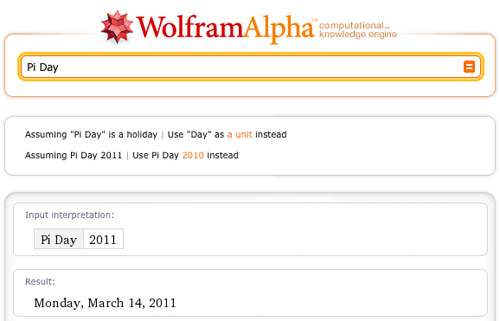…and, in the process, swap trivia of note on everyone’s (including Wolfram|Alpha’s) favorite number.

There have been no shortage of blog posts already written on the subject; see, for example, last year’s “Pi Day in Wolfram|Alpha” (or the Wolfram Blog Pi Day posts from 2008 or 2010). As already hinted at in last year’s blog, one would expect the pi to be ubiquitous in a computational knowledge engine—and so it is. Therefore, at the risk of beating a proven transcendental constant to death, this year we offer a few (well, OK: more than a few) additional pi-related esoterica courtesy of Wolfram|Alpha.

Some of the more prosaic (read: direct mathematical) occurrences of pi include in the circumference of a circle, total surface area of a cylinder, surface area of a torus, and volume of an n-dimensional sphere:Also on the mathematical side of pi, there are many ways to express rational multiples of pi as sums of inverse cotangents using so-called Machin-like formulas:Many sums and integrals result in pi as well, for example:
sum 4 (-1)^k/(2 k + 1) from k = 0 to infinity, sum 2 (2 k – 1)!!/((2 k + 1) (2 k)!!) from k = 0 to infinity, integrate 4 sqrt(1-t^2) from t = 0 to 1, and integrate 8/3 sin^3t/t^3 from t = 0 to infinity.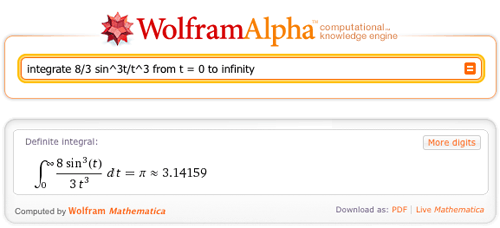Pi occurs as the limit of various mathematical expressions, such as limit 2^(4 n)/(n binomial (2 n, n)^2) as n -> inf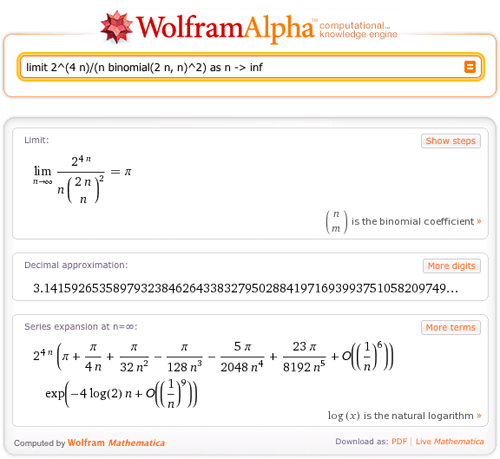…and also as the value of many special functions at certain significant points, including 2 arcsin(1), 2 ellipticK(0), gamma (1/2)^2: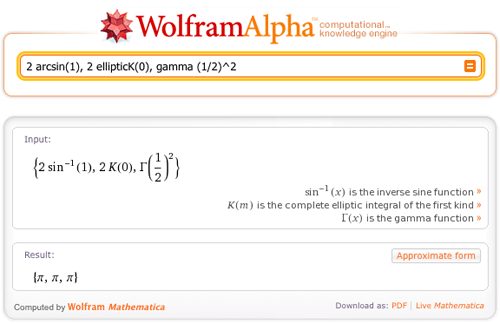Finding out more about pi is easy with Wolfram|Alpha: pi to 1000 digits, pi in base 314, identities for pi, and so on. Furthermore, Wolfram|Alpha is able to recognize possible closed forms for approximate numerical input, so given a decimal approximation of pi, we can recover the constant (along with other possible closed forms); try, for example, 3.1415 or 3.141592:(Note that in the case of the latter input, the “other” possible closed forms are in fact exactly equal to pi through mathematical identities.)

Along similar lines, Wolfram|Alpha can express a given number through pi; for example, express 10. through pi and pi!:OK, so much for mathematical esoterica of pi. What is less well-known (and deserves to be known better!) is how often pi occurs “naturally” in day-to-day, real-world situations when relevant properties are measured in suitable pi-adapted units. We can, for example, use the power of Wolfram|Alpha to observe pi in the length of the Tagus River expressed in sus: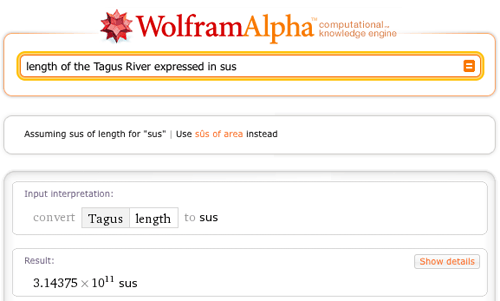Here are a selection of other inputs that highlight the significance of pi in the properties of natural and man-made objects, physical phenomena, and various other human endeavors:

Deciding if a value is related to pi can be more tricky for time-varying phenomena, but we see it at least in current data for the circulation Western Outdoor News + circulation Golf Vacations magazine + circulation American Baby’s First Year of Life: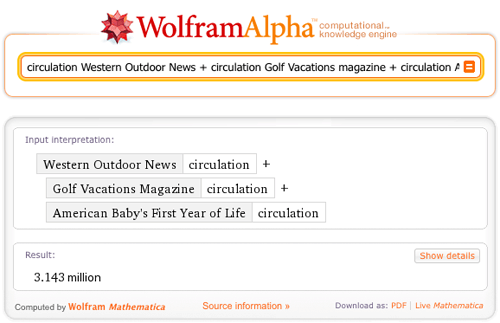…as well in a selection of other such inputs:

While pursuing these observations, we encourage the user to have fun, but also to be aware that some of the above results might just exceed an arbitrary threshold of say, approximately pi canards, if their scientific significance were taken too seriously.

Finally, it is good so see that while pi remains irrational, this level-headed constant still does not let all the festivities on its behalf interfere with its usual daily routine. 😉

Data for this post was compiled by Michael Trott.

Greetings Eric & Michael

I read Jonathan M. Borwein’s ‘The Life of Pi: History and Computation: a Talk for PiDay’, all 339 pages (piday.pdf) and it has taken me a number of days and I’m still not to the end!

I have decided brevity is the key word!

Best regards,

George

Posted by George March 14, 2011 at 2:41 pm

May I ask why July 22nd ( 7/22) is not pi day, since days and months are measured in counting numbers and 3.14 is a decimal? I have been doing some funky things with the 22 divided by 7 phenomenon besides calculating it to ridiculously large decimal places. There’s way more to pi than just an irrational number. When it is still expressed as something that resembles a ratio it has some very unique abilities to inform geometry from a visual and construction point of view. The result is Gravity/sphere theory and a whole new look at a lot of things that are not quite round enough to qualify for pi. Why is nobody talking about gravity/sphere theory on July 22nd, hum? :-))))))

Sincerely, Lost in Irrational Outer Space., Michael

Posted by Michael Greedley March 15, 2011 at 6:47 pm

of course Pi day is only on 14 March if you uses the US calendar which places the month before the day and then adds the year (which as Spock would say – is just not logical).

In countries where the date is ddmmyy (finest to coarsest granularity) we are just unfortunate to not have one 🙂

Posted by Rae March 16, 2011 at 1:30 am

Let’s say I knew that there’s this nice relationship between pi, i, and e, but I had forgotten the exact formula.

Reading above “express 10. through pi and pi!” example, I would then try phrasing

“express pi through e and i” which W|A interprets as a simple “pi”
“express pi through the constants e and i” tells me about a first name of “Constant”
“express -1 through e and i and pi” which W|A doesn’t understand at all
“express -1. through e and i and pi” shows some relationships, including the funny statement that -1 is an approximation of (and not equal to) -1.00000000)

None of the above will reveal that e^(-i pi) = -1

My experience is, that in general it’s so difficult to find the correct phrasing for a problem (even, as above, if you’ve got a working example to start from). Not sure if or how any of the following might help: 1) more documentation for the current W|A input language, or 2) a new (optional) formalized and fully documented input language, or 3) W|A asking back questions on how to interpret any phrase you’ve entered

Posted by ErichSt March 16, 2011 at 4:56 am

Great job! Pi is so cool.

Posted by Mimi March 22, 2011 at 3:43 pm